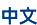## Uncertainty Quantification

Uncertainty is everywhere. In order to quantify the uncertainty of mathematical models for practical problems and reduce the risks caused by uncertainty, a cross-research field Uncertainty Quantification (UQ) has emerged in academia and industry across the world, modeling and simulating  practical problems with uncertainty numerically. Uncertainty quantification is a hot research topic in recent years, and has a variety of applications, including hydrology, fluid mechanics, data assimilation, and weather forecasting.

Polynomial chaos (PC) methods are one of the most widely used computational methods for stochastic mathematical models in recent years.

The main research interests of our research group include algorithm design for high-order numerical methods of uncertainty quantification, regularity analysis and error analysis, and applications to hyperbolic conservation law equations and inverse problems with random inputs.

• 简介

Uncertainty is everywhere. In order to quantify the uncertainty of mathematical models for practical problems and reduce the risks caused by uncertainty, a cross-research field Uncertainty Quantification (UQ) has emerged in academia and industry across the world, modeling and simulating  practical problems with uncertainty numerically. Uncertainty quantification is a hot research topic in recent years, and has a variety of applications, including hydrology, fluid mechanics, data assimilation, and weather forecasting.

Polynomial chaos (PC) methods are one of the most widely used computational methods for stochastic mathematical models in recent years.

The main research interests of our research group include algorithm design for high-order numerical methods of uncertainty quantification, regularity analysis and error analysis, and applications to hyperbolic conservation law equations and inverse problems with random inputs.